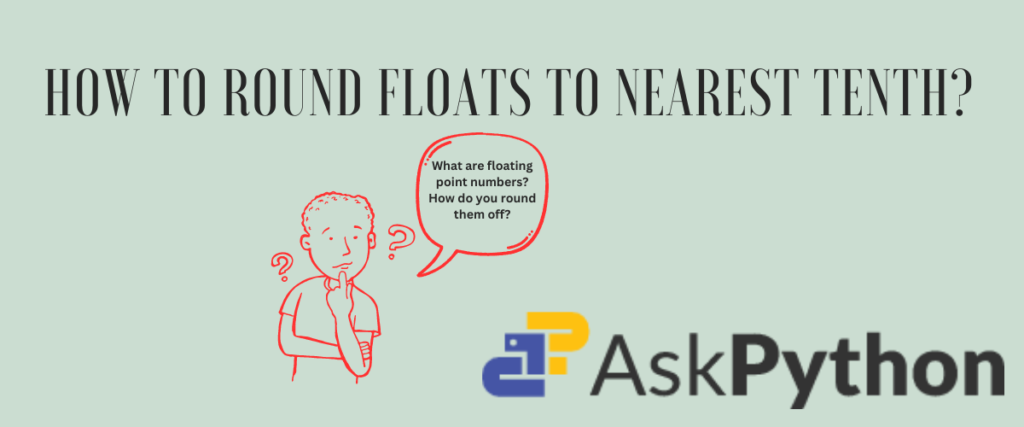# 2 Ways to Round a Float to Nearest 10thA floating-point number( shortened as float) is any positive or negative, whole or fractional number that has a decimal point. Sometimes, even a number without a decimal point can be treated as a floating-point number. 3.145, 2.3×10-2, and so on are a few examples.

The data type that is associated with such numbers is known as float. There is an interesting concept behind the naming of floating-point numbers. You see, the decimal point in such numbers is not fixed. The decimal point moves according to the power of the exponent. The same number may have different decimal positions with different exponents. Since the decimal point moves with respect to the exponent, they are called floating-point numbers.
Consider the number 2.344. If this number is dealt with an exponent of 10-2 which is 2.344×10-2, the result is 0.02344. Whereas 2.344×102 results in 234.4. So with the change in the exponents, the decimal point also moves accordingly.

The scientific notation for storing huge floats is to use the exponents that were discussed in the above example. However, we don’t always need the entire floating-point number.

Learn how to suppress the scientific notation of float values here

The float datatype holds significance in most programming languages. In languages like C, Java, and Python, the floating point numbers are all grouped into a datatype called float, whereas a few languages like JavaScript use the Float datatype as a hood for all the numbers, including integers internally.

Let us understand floating-point numbers and how to round them to the nearest 10th with the help of a few examples.

## What Are Floating Point Numbers?

Floating point numbers are those representations that support decimal points. Let us see a few examples.

```print("Example 1")
fnum = 0.0023
print("Example of floating point number:",fnum)
print("The data type of",fnum, "is:", type(fnum))
```

In line 2, we are assigning a number to the variable fnum. Then we print the number on the screen. The last print statement is used to display the class of the number.

Now that we have understood what floating point numbers are, let us try to convert an integer to a float.

```print("Conversion of integer to float")
intnum1 = 245
intnum2 = -12
print("The integer values are:",intnum1,intnum2)
fnum1 = float(intnum1)
fnum2 = float(intnum2)
print("The respective float values are:",fnum1,fnum2)
```

In the second and third lines, we are assigning two integers to `intnum1` and `intnum2`. Next, we are printing them. Next, we are converting these integers to float using the method `float`. Lastly, we are printing the converted float values.

We can check the data types of the variables before and after conversion using `type()` method.

```print(intnum1,":", type(intnum1))
print(intnum2,":", type(intnum2))
print(fnum1,":", type(fnum1))
print(fnum2,":", type(fnum2))
```

## How to Round the Float Values to Nearest 10th?

While floating point numbers are useful in many fields, including scientific calculations, representing large numbers, and even bit manipulation, we do not always need the entire float range after the decimal point. We just need two digits after the decimal point to continue with our tasks. Rounding off may be useful in such cases.

In our case, rounding off the number to the nearest tenth digit results in one digit after the decimal point.

We all studied rounding numbers in high school mathematics, but it is indeed important to recapitulate.
To round off a number to its 10th position, we check if the number after it (the digit in the hundredth place) is greater than or equal to 5. If it is so, we add one to the number in the tenth digit. If the hundredth digit is not greater than 5, well, we don’t do anything! The number in the tenth place is retained as it is.

### Using the Round Function

The round function in Python is used to round the digit to the given number of digits after the decimal point. It takes the number to be rounded off and the precision digit.

The syntax is as follows:

```round(number, ndigits=None)
```

The n-digits is used to specify how the round function should work. We will see an example of using the round function to round off a floating point number.

def round_to_10(num):
return round(num,1)
num = float(input(“Enter a float value of your choice:”))
rnum = round_to_10(num)
print(rnum)

A user-defined function called round_to_10 which rounds the floating point number to its nearest 10th digit using the round function.
We are asking the user to enter a number of their choice to round it off.

The result is stored in a variable called rnum which is printed on the next line.

### Using the Format Specifier

The format specifiers work very well with strings, but we can also use these specifiers to round the floating point numbers to the precision digit we wish.

Understand %.3f formatting here

Similar to the previous example, we are going to create a special function defined by us to round the floating point number. Follow the code below.

num1 = float(input(“Enter a float value of your choice:”))
def round_to_10f(num1):
return “{:.1f}”.format(num1)
rfnum = round_to_10f(num1)
print(rfnum)

We are taking input from the user that is stored in the variable called num1. With the help of `def` keyword, we have created a function called round_to_10f. In this function, we are using 1f to print the nearest 10th digit of the float input. The result is stored in a variable called rfnum.